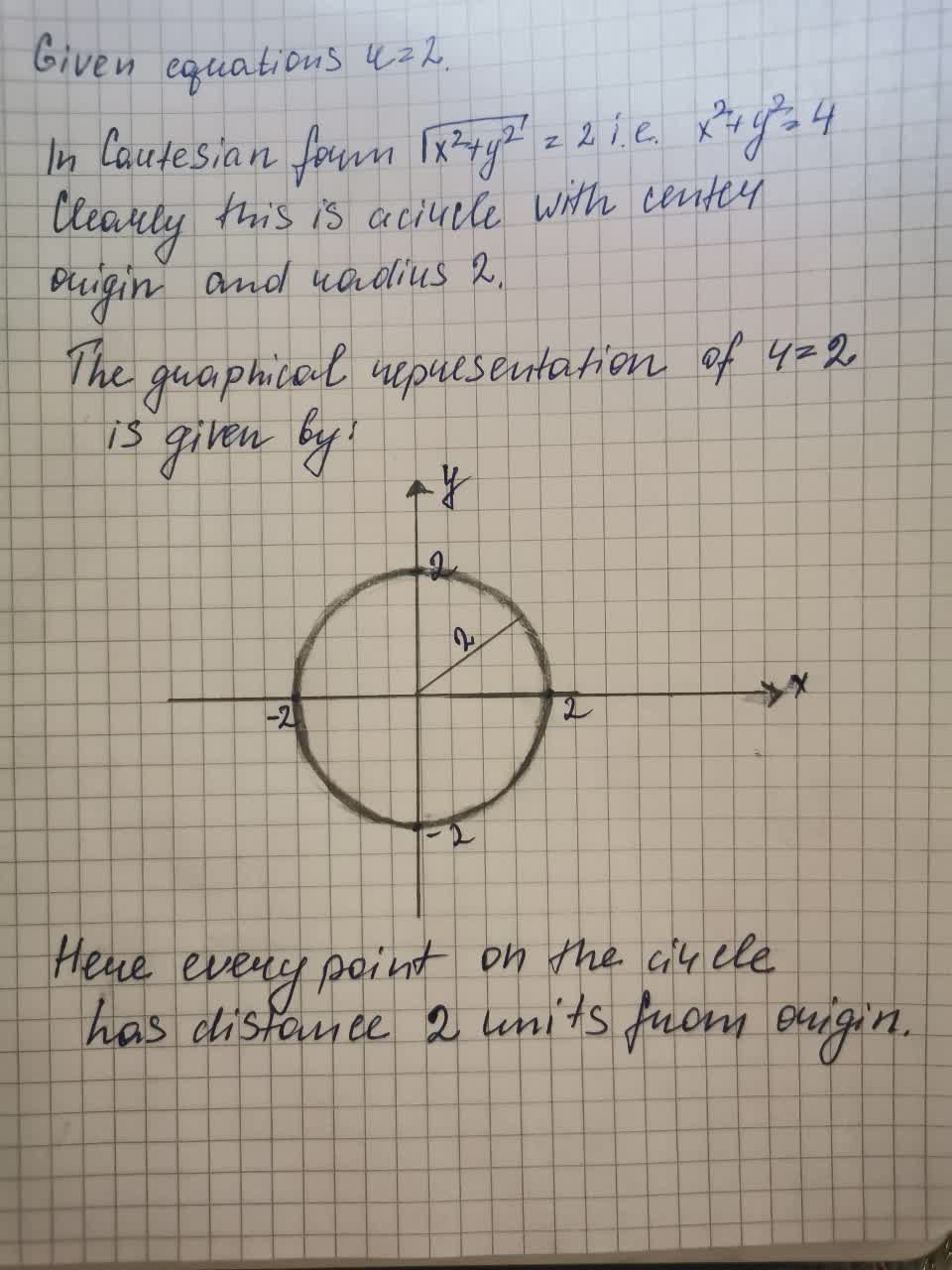Graph the sets of points whose polar coordinates satisfy the equations and inequalities rBrennan Flores 2021-08-20 Answered
Graph the sets of points whose polar coordinates satisfy the equations and inequalities r = 2

• Questions are typically answered in as fast as 30 minutes

Solve your problem for the price of one coffee

• Math expert for every subject
• Pay only if we can solve itgrbavit

to satisfy the equations